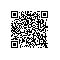# 从原理到代码，轻松深入逻辑回归模型！

【导语】学习逻辑回归模型，今天的内容轻松带你从0到100！阿里巴巴达摩院算法专家、阿里巴巴技术发展专家、阿里巴巴数据架构师联合撰写，从技术原理、算法和工程实践3个维度系统展开，既适合零基础读者快速入门，又适合有基础读者理解其核心技术；写作方式上避开了艰涩的数学公式及其推导，深入浅出。

## 0、前言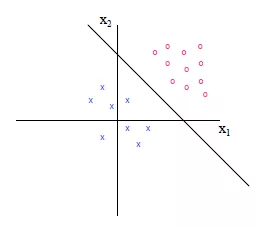## 1、Sigmoid 函数

Sigmoid 的函数表达式如下：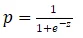import numpy as np
import matplotlib.pyplot as plt

def sigmoid(x):
y = 1.0 / (1.0 + np.exp(-x))
return y

plot_x = np.linspace(-10, 10, 100)
plot_y = sigmoid(plot_x)
plt.plot(plot_x, plot_y)
plt.show()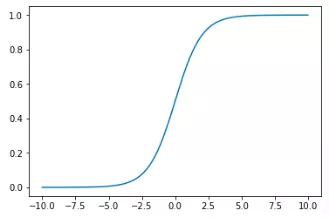## 2、 梯度下降法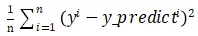import numpy as np
import matplotlib.pyplot as plt
if __name__ == '__main__':
plot_x = np.linspace(-1, 6, 141) #从-1到6选取141个点
plot_y = (plot_x - 2.5) ** 2 – 1 #二次方程的损失函数
plt.scatter(plot_x, plot_y, color='r') #设置起始点，颜色为红色
plt.plot(plot_x, plot_y)
# 设置坐标轴名称
plt.xlabel('theta', fontproperties='simHei', fontsize=15)
plt.ylabel('损失函数', fontproperties='simHei', fontsize=15)
plt.show()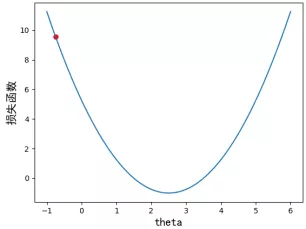1. 首先定义损失函数及其导数
def J(theta): #损失函数
return (theta-2.5)**2 -1

def dJ(theta): #损失函数的导数
return 2 * (theta - 2.5)
1. 通过 Matplotlib 绘制梯度下降迭代过程，具体代码如下：
theta = 0.0 #初始点
theta_history = [theta]
eta = 0.1 #步长
epsilon = 1e-8 #精度问题或者eta的设置无法使得导数为0
while True:
last_theta = theta #先记录下上一个theta的值
theta = theta - eta * gradient #得到一个新的theta
theta_history.append(theta)
if(abs(J(theta) - J(last_theta)) < epsilon):
break #当两个theta值非常接近的时候，终止循环
plt.plot(plot_x,J(plot_x),color='r')
plt.plot(np.array(theta_history),J(np.array(theta_history)),color='b',marker='x')
plt.show()      #一开始的时候导数比较大，因为斜率比较陡，后面慢慢平缓了
print(len(theta_history)) #一共走了46步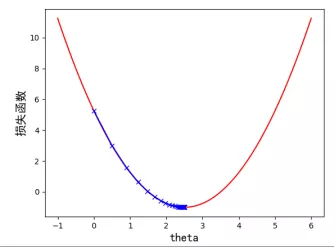## 3、学习率的分析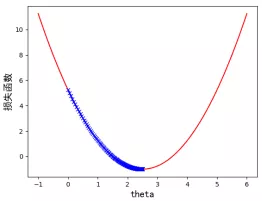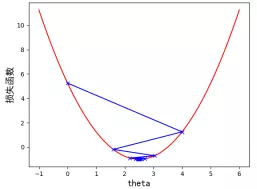def J(theta):
try:
return (theta-2.5)**2 -1
except:
return float('inf')

i_iter= 0
n_iters = 10
while i_iter < n_iters:
last_theta = theta
theta = theta - eta * gradient
i_iter += 1
theta_history.append(theta)
if (abs(J(theta) - J(last_theta)) < epsilon):
break # 当两个theta值非常接近的时候，终止循环

## 4、逻辑回归的损失函数

import numpy as np
import matplotlib.pyplot as plt

def logp(x):
y = -np.log(x)
return y

plot_x = np.linspace(0.001, 1, 50) #取0.001避免除数为0

plot_y = logp(plot_x)
plt.plot(plot_x, plot_y)
plt.show()import numpy as np
imort matplotlib.pyplot as plt

def logp2(x):
y = -np.log(1-x)
return y

plot_x = np.linspace(0, 0.99, 50) #取0.99避免除数为0
plot_y = logp2(plot_x)
plt.plot(plot_x, plot_y)
plt.show()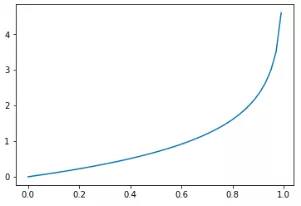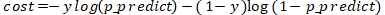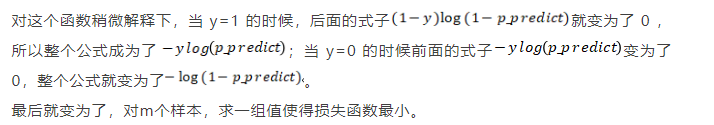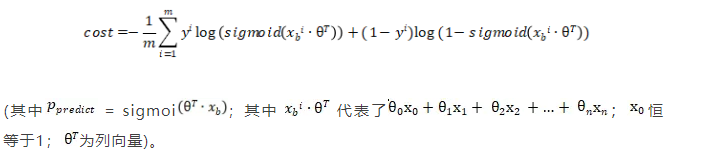## 5、Python实现逻辑回归

class LogisticRegressionSelf:

def __init__(self):
"""初始化Logistic regression模型"""
self.coef_ = None #维度
self.intercept_ = None #截距
self._theta = None


Sigmoid 函数那个小节已经实现过了，对于这个函数我们输入的值为多元线性回归中的（其中恒等于1），为了增加执行效率，我们建议使用向量化来处理，而尽量避免使用 for 循环，所以对于我们使用来代替，具体代码如下：

    def _sigmoid(x):
y = 1.0 / (1.0 + np.exp(-x))
return y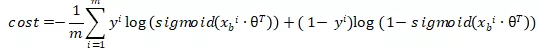#计算损失函数
def J(theta,X_b,y):
p_predcit = self._sigmoid(X_b.dot(theta))
try:
return -np.sum(y*np.log(p_predcit) + (1-y)*np.log(1-p_predcit)) / len(y)
except:
return float('inf')

import numpy as np

class LogisticRegressionSelf:

def __init__(self):
"""初始化Logistic regression模型"""
self.coef_ = None #维度
self.intercept_ = None #截距
self._theta = None

#sigmoid函数，私有化函数
def _sigmoid(self,x):
y = 1.0 / (1.0 + np.exp(-x))
return y

def fit(self,X_train,y_train,eta=0.01,n_iters=1e4):
assert X_train.shape == y_train.shape, '训练数据集的长度需要和标签长度保持一致'

#计算损失函数
def J(theta,X_b,y):
p_predcit = self._sigmoid(X_b.dot(theta))
try:
return -np.sum(y*np.log(p_predcit) + (1-y)*np.log(1-p_predcit)) / len(y)
except:
return float('inf')

#求sigmoid梯度的导数
def dJ(theta,X_b,y):
x = self._sigmoid(X_b.dot(theta))
return X_b.T.dot(x-y)/len(X_b)

#模拟梯度下降
theta = initial_theta
i_iter = 0
while i_iter < n_iters:
last_theta = theta
theta = theta - eta * gradient
i_iter += 1
if (abs(J(theta,X_b,y) - J(last_theta,X_b,y)) < epsilon):
break
return theta

X_b = np.hstack([np.ones((len(X_train),1)),X_train])
initial_theta = np.zeros(X_b.shape) #列向量
self.intercept_ = self._theta #截距
self.coef_ = self._theta[1:] #维度
return self

def predict_proba(self,X_predict):
X_b = np.hstack([np.ones((len(X_predict), 1)), X_predict])
return self._sigmoid(X_b.dot(self._theta))

def predict(self,X_predict):
proba = self.predict_proba(X_predict)
return np.array(proba > 0.5,dtype='int')


## 小结